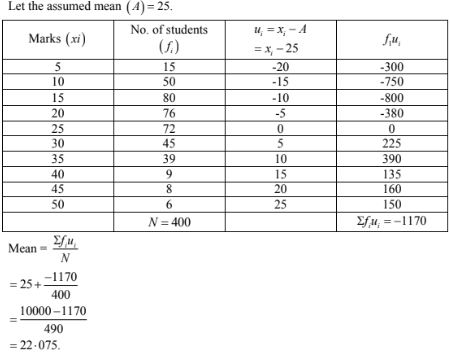# RD Sharma Solutions Chapter 7 Statistics Exercise 7.2 Class 10 Maths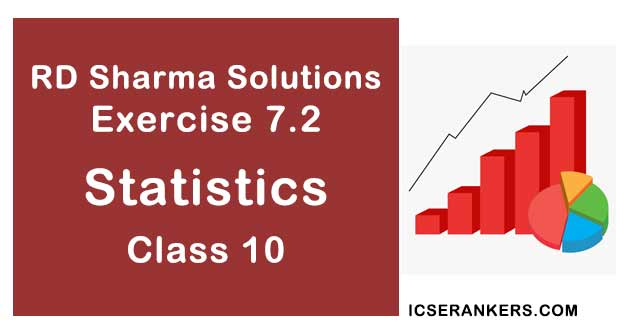Chapter Name RD Sharma Chapter 7 Statistics Book Name RD Sharma Mathematics for Class 10 Other Exercises Exercise 7.1Exercise 7.3Exercise 7.4Exercise 7.5Exercise 7.6 Related Study NCERT Solutions for Class 10 Maths

### Exercise 7.2 Solutions

1. The number of telephone calls received at an exchange per interval for 250 successive one - minute intervals are given in the following frequency table :

 No. of calls (x) 0 1 2 3 4 5 6 No. of intervals (f) 15 24 29 46 54 43 39
Compute the mean number of calls per interval.

Solution

Let be assumed mean (A) = 3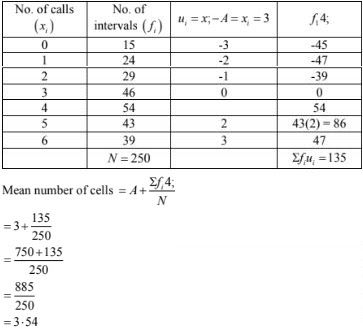2. Five coins were simultaneously tossed 1000 times, and at each toss the number of heads was observed. The number of tosses during which 0,1,2,3,4 and 5 heads were obtained are shown in the table below. Find the mean number of heads per toss
 No. of heads per toss(x): 0 1 2 3 4 5 No. of tosses (f): 38 144 342 287 164 25

Solution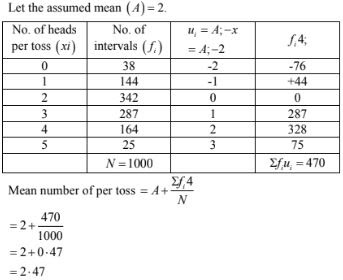3. The following table gives the number of branches and number of plants in the garden of a school.
 No. of branches (x): 2 3 4 5 6 No. of plants(f): 49 43 57 38 13

Calculate the average number of branches per plant.

Solution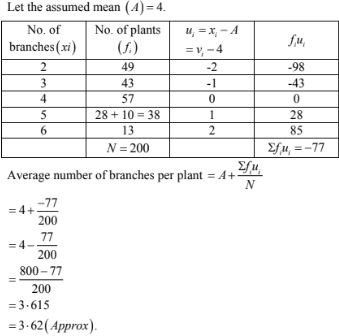4. The following table gives the number of children of 150 families in a village
 No. of children(x): 0 1 2 3 4 5 No. of families(f): 10 21 55 42 15 7

Solution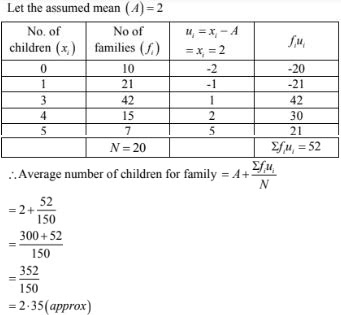5. The marks obtained out of 50, by 102 students in a physics test are given in the frequency table below:
 Marks(x): 15 20 22 24 25 30 33 38 45 Frequency(f): 5 8 11 20 23 18 13 3 1

Find the average number of marks.

Solution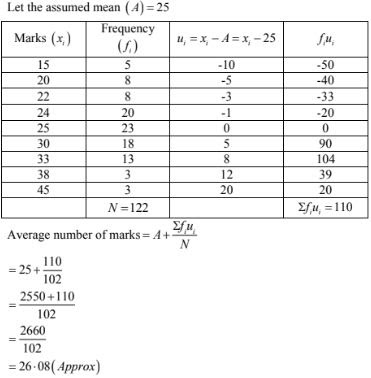6. The number of students absent in a class were recorded every day for 120 days and the information is given in the following frequency table:
 No. of students absent(x): 0 1 2 3 4 5 6 7 No. of days(f): 1 4 10 50 34 15 4 2

Find the mean number of students absent per day.

Solution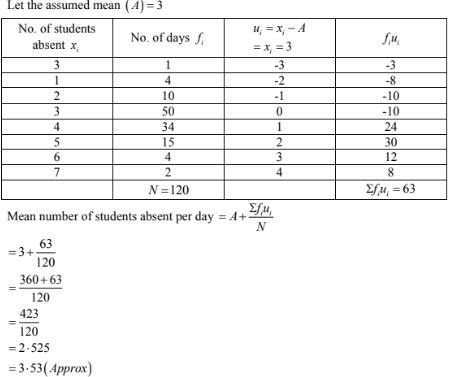7. In the first proof reading of a book containing 300 pages the following distribution of misprints was obtained:
 No. of misprints per page (x) 0 1 2 3 4 5 No. of pages(f); 154 95 36 9 5 1

Find the average number of misprints per page.

Solution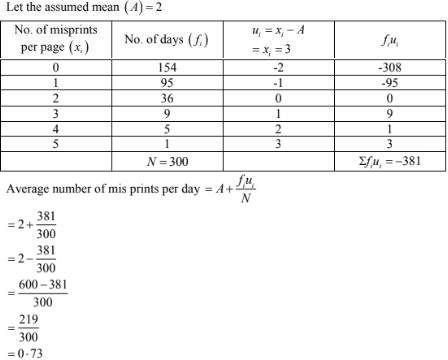8. The following distribution gives the number of accidents met y 160 workers in a factory during a month.
 No. of accidents(x): 0 1 2 3 4 No. of workers(f): 70 52 34 3 1

Find the average number of accidents per worker.

Solution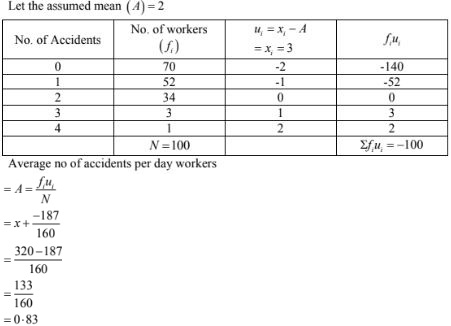9. Find the mean from the following frequency distribution of marks at a test in statistics:
 Marks(x): 5 10 15 20 25 30 35 40 45 50 No. of students(f): 15 50 80 76 72 45 39 9 8 6

Solution